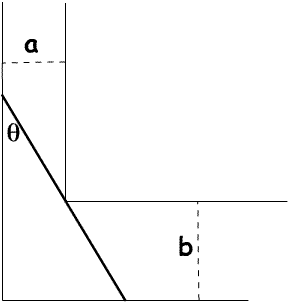#### You may also like### Generally Geometric

Generalise the sum of a GP by using derivatives to make the coefficients into powers of the natural numbers.### Exponential Trend

Find all the turning points of y=x^{1/x} for x>0 and decide whether each is a maximum or minimum. Give a sketch of the graph.### Slide

This function involves absolute values. To find the slope on the slide use different equations to define the function in different parts of its domain.

# Bend

##### Age 16 to 18Challenge LevelA long stick has to be carried horizontally along a narrow corridor and around a right-angled bend. The corridor is $a$ centimetres wide on one side of the bend and $b$ centimetres wide on the other side. Find, in terms of $a$ and $b$, the length of the longest stick that can be manoeuvered horizontally around the bend?

If the object is $2$ metres long and the two branches of the corridor are $65$ centimetres and $75$ centimetres respectively is it possible to manoeuvre the stick around the bend?